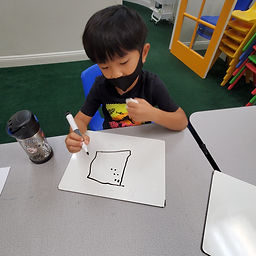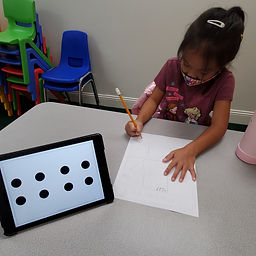## Ms. Raejean

### Target 1​

###### Lesson Type:

New

Number Operation

:

Number Meaning

Match number and quantity for numbers zero to 100.

###### 1:

Count objects to determine how many there are.

###### 2:

Recognize the quantities zero to ten, without having to count.

###### 3:

Develop strategies of grouping quantities to count larger quantities faster (i.e. base-ten blocks).

2nd

###### Vocabulary:

Pattern, Grouping

Activities:

Students were shown "Quick Images" of dots. Each card was shown twice for about 3 seconds each. The goal was for students to tell how many dots were in the picture by seeing patterns and grouping the dots instead of having to count each dot. The first images were easier for students to count the dots. Students drew what they saw for other images and verbalized how they counted the dots. (Ex. 6 ~ 1, 2, 3, 4, 5, 6 OR 7 ~ 2 & 5)### Home Exploration

###### Guiding Questions:## Absent Students:

### Target 2

:

###### 1:

Identify the Commutative Property of Addition.

###### 2:

Understand that changing the order of addends, does not change the sum.

2nd

###### Vocabulary:

Sum, Turn Around (change the order)

Activities:

• Students used a scale and counting bears to add quantities. [Ex. On 1 side - Add 3 red bears and 5 purple bears. On the 2nd side - Add 5 purple bears and 3 red bears.] After a few rounds, students were asked, "What do you notice about the numbers we added?" We discovered that when we added the numbers, then changed the order, we still got the same answer.
• Next, students used dominoes to continue to explore how we can turn around the numbers in an addition sentence, but still get the same answer. Students recorded the dots on each domino, turned it around, recorded the dots in their "new" position, and found the sum of the addition sentences.
• We talked about starting with the larger number and adding on the smaller number. [Ex. 2 + 4 = 4 + 2. Start with 4 and count on, so 4, 5, 6.]### Home Exploration

###### Guiding Questions:### Target 3

:

###### Vocabulary:

Activities:### Home Exploration